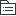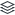# MySQL函数

149
|
21

## 相关模板推荐

•## mysql函数 —— 作品大纲

•数学函数
• abs()返回绝对值
• sign(x)返回x的符号，负数为-1,正数为1，0
• bin()返回二进制
• oct()返回八进制
• hex()返回十六进制
• exp(x)返回e的x次方
• ln(x)返回x的自然对数
• log(x,y)返回以x为底，y的对数
• sqrt(x)返回x的平方根
• truncate(x,y)返回x直接截取前y位小数的结果
• mod(x,y)返回x/y的商，为四舍五入的整值
• ceiling(x)大于x的最小整数
• floor(x)小于x的最大整数
• greatest(x1,x2,x3...)返回数集中的最大值
• least(x1,x2,x3,...)返回数集中的最小值
• pi()返回圆周率
• rand()返回0—1内的随机值
• round(x,y)返回x四舍五入保留y位小数的值
•聚合函数
• avg()返回指定列的平均值
• sum()返回指定列的和
• max()返回指定列的最大值
• min()返回指定列的最小值
• count(*)返回所有列包括空行的行数
• count(列名)返回指定列非空行数
• group_concat()返回由属于一组的列值连接组合而成的结果
•字符串函数
• ASCII(str)返回字符串的ASCII码值
• length(str)返回字符串字节长度
• bit_length()返回字符串比特长度
• char_length()返回字符串字符长度
• concat(s1,s2,s3...)将字符串连接起来
• concat_ws(s,s2,s3,...)将字符串连接起来，并用sep字符间隔，第一个字符为分隔符
• position(substr in str)返回子串substr在字符串str中第一次出现的位置
• find_in_set(str,list)分析逗号分隔的list列表，如发现str，返回str在list中的位置
• insert(str,x,y,instr)返回字符串，从第x个位置开始，y个字符长的子串替换为字符串instr
• lcase()或lower()返回字符串中所有字符变小写的结果
• ucase()或upper()返回字符串中所有字符变大写的结果
• left(str,x)返回字符串中最左边的x个字符
• right(str,x)返回字符串右边x个字符
• ltrim(str)从字符串中切掉开头的空格
• rtrin()返回字符串去掉尾部的空格
• trim(str)去除首部和尾部的空格
• quote(str)用反斜杠转义str中的单引号
• repeat(str,x)将str重复x次
• reverse(str)将字符串反转
• strcmp(s1,s2)比较字符串s1和s2,相等返回0，小于返回-1，大于返回1
•日期和时间函数
• curdate()或current_date()返回当前日期
• curtime()或current_time()返回当前时间
• now()返回当前日期和时间
• date_format(date,fmt)依照制定的fmt格式格式化日期date值
• from_unixtime(ts,fmt)根据制定的fmt格式，格式化unix时间戳ts
• date_sub(date,interval int keyword)返回日期date减去时间间隔int的结果
• dayofweek(date)返回日期是一周中的第几天
• dayname()返回日期所在的星期名
• dayofmonth()返回date是一个月中的第几天
• dayofyear()返回日期代表的是一年中的第几天
• hour(time)返回时间的小时值
• minute(time)返回时间的分数值
• year(date)返回日期的年份
• month(date)返回日期的月份值，第几个月
• monthname(date)返回日期的月份名
• quarter(date)返回日期为一年中第几季度
• week(date)返回日期为一年中第几周
•加密函数
• encode(str,key)使用key作为密钥加密字符串str，调用encode()的结果是一个二进制字符串存储
• decode()
• md5()
• sha()
•控制流函数
• case 列名 when 条件1 then 结果1 when 条件2 then 结果2 ...else 结果;
• if(tesr,t,f)如果test为真，返回t，否则返回f
• ifnull(arg1,arg2)如果arg1不空，返回arg1,否则返回arg2
• nullif(arg1,arg2)如果arg1和arg2相等，返回null，否则返回arg1
•系统调试函数
• user()返回用户及所在主机，判断自己身份
• database()返回当前正在操作的库名
• version()返回版本
•格式化函数
• date_format(date,fmt)依照字符fmt格式化日期值
• format(x,y)把x格式化以逗号隔开的数字序列，y是结果的小数位数
• inet_aton(ip)返回ip地址的数字表示
• inet_ntoa(num)返回数字所代表的ip地址
• time_format(time,fmt)依照字符串fmt格式化时间time值
•类型转化函数
• cast()把一个值转化为指定的数据类型，类型有：binary,char,date,time,datetime,signed,unsigned